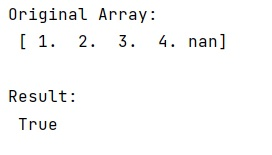# Fast check for NaN in NumPy

Learn, how to check for NaN in NumPy?
Submitted by Pranit Sharma, on December 26, 2022

NumPy is an abbreviated form of Numerical Python. It is used for different types of scientific operations in python. Numpy is a vast library in python which is used for almost every kind of scientific or mathematical operation. It is itself an array which is a collection of various methods and functions for processing the arrays.

## Checking for NaN in NumPy

While creating a DataFrame or importing a CSV file, there could be some NaN values in the cells. NaN values mean "Not a Number" which generally means that there are some missing values in the cell.

We need to look for the fastest way to check for the occurrence of NaN in a NumPy array.

A good solution for this purpose is to use the numpy.isnan() inside which we will use numpy.min() method.

Let's understand with the help of an example,

## Python code to fast check for NaN in NumPy

```# Import numpy
import numpy as np

# Creating a numpy array
arr = np.array([1,2,3,4,np.nan])

# Display original array
print("Original Array:\n",arr,"\n")

# Check for nan
res = np.isnan(np.min(arr))

# Display result
print("Result:\n",res,"\n")
```

Output: